Saturday, November 28, 2020
Home > Latest Announcement > NMTC PREVIOUS YEAR PAPERS WITH SOLUTIONS

# NMTC PREVIOUS YEAR PAPERS WITH SOLUTIONS# NMTC Previous Year Papers with Solutions

NMTC is a short form of National Mathematics Talent Competition. This is conducted by AMTI to identify the talent of the students in Mathematics. The students who have an ability for unique creativity thinking a unique and imaginative considering, preparation to assault new and non-routine issues displaying a general numerical capacity proper to their level. In this post, you will get NMTC Previous Year Papers with Solutions according to class wise.

Amans Maths Blogs (AMB) created an ebook of NMTC Previous Year Papers with Solutions according to class wise: for Primary Level (Class 5 & 6), for Sub-Junior Level (Class 7 & 8) and Junior Level (Class 9 & 10). These books are the alternative and best books for NMTC preparation, because previous year questions papers with answer keys and solutions will give an idea of NMTC syllabus and their difficulty level of asked questions in NMTC question paper or NMTC past papers. In this post, you will get an ebook of NMTC Stage 1 and 2 Primary Level Previous Year Papers Solutions PDF.

AMTI is a short form of Association Mathematics Teachers of India. It is an academic body of professionals and students who have a passion in Mathematics research and education. For the AMTI NMTC exam, you need amti previous question papers with solutions. NMTC exams are in two stages. It is called as NMTC Stage 1 and Stage 2 or NMTC Screening Level and NMTC Final Level exam.

According to AMTI, the students who have an ability for unique creativity thinking a unique and imaginative considering, preparation to assault new and non-routine issues displaying a general numerical capacity proper to their level. they must start the preparation of NMTC exam. For the NMTC preparation, you need NMTC study material, or you can also say it as AMTI exam books for NMTC.

To clear NMTC exam, you need NMTC Papers with Solutions it will help you in the preparation of this exam, So I created an EBook of NMTC Previous Question Papers with Solutions PDF.

Now, for more details about the NMTC exam, like its NMTC eligibility, medium of NMTC papers, examination fee, and NMTC syllabus etc, click here : NMTC Notification

## NMTC Stage 1 Primary Level Previous Years Questions Papers with Solutions

NMTC exams is categorized into five levels according to class/grade studying the students. The students may be from Schools, Junior Colleges of all boards, Degree Colleges and Technological Institutions in India and also Schools affiliated to the CBSE abroad. NMTC Primary Level exam is known as Gauss Contest, its eligibility is for Class 5 and 6. Thus, to prepare for NMTC Primary level exam, you need NMTC Stage 1 Primary Level Previous Years Questions Papers with Solutions.

### NMTC 2004 Stage 1 Primary Level Questions Papers With Solutions

Question 1 :

Look at the following dot diagram.The pattern continues. The value of 1 + 3 + 5 +…. + up to 100 terms is the number of dots shown in the

(A) 100th diagram and the number of dots present in it is 1000

(B) 1000th diagram and the number of dots present in it is 10000

(C) 100th diagram and the number of dots present in it is 10,000

(D) 1000th diagram and the number of dots present in it is 1000

Solution 1 :Question 2 :

Look at the rows of the numbers shown below.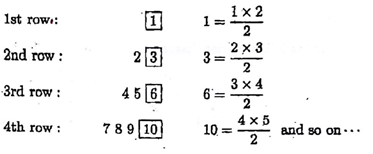The first number in the 50th row is

(A) 1275

(B) 1224

(C) 1276

(D) 1226

Solution 2 :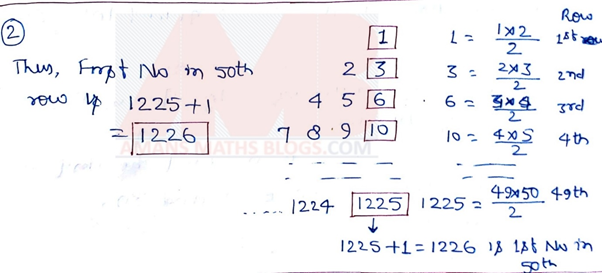### NMTC 2005 Stage 1 Primary Level Questions Papers With Solutions

Question 1 :

The date of the 100th day of any year that is not a leap year is

(A) April 10

(B) April 2

(C) April 7

(D) April 9

Solution 1 :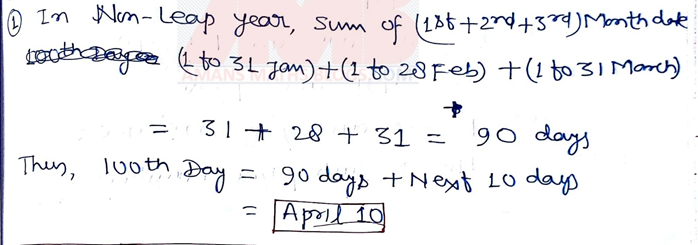Question 2 :

The number of two digit numbers of the form aa, with the same digits ‘a’ having exactly four divisors is

(A) 2

(B) 4

(C) 6

(D) 8

Solution 2 :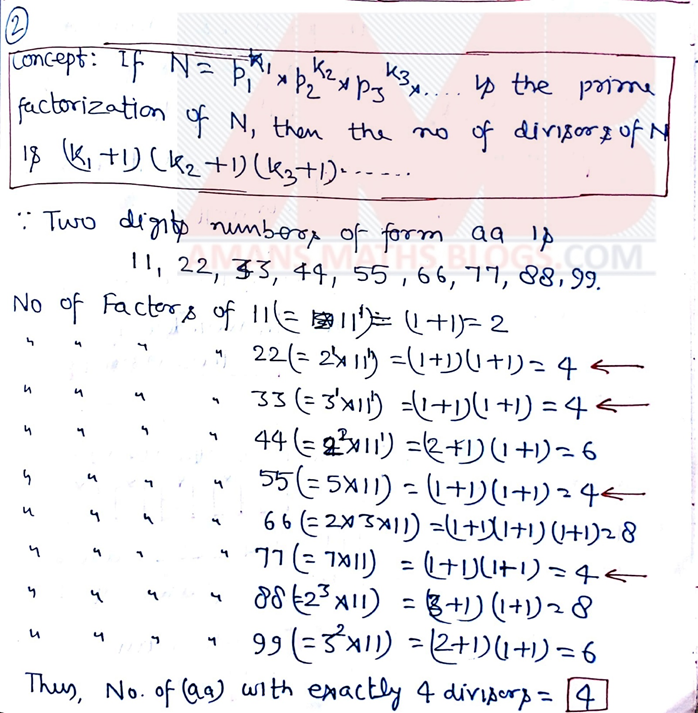### NMTC 2006 Stage 1 Primary Level Questions Papers With Solutions

Question 1 :

The number of 3 digit numbers which end in 7 and are divisible by 11 is

(A) 2

(B) 4

(C) 6

(D) 8

Solution 1 :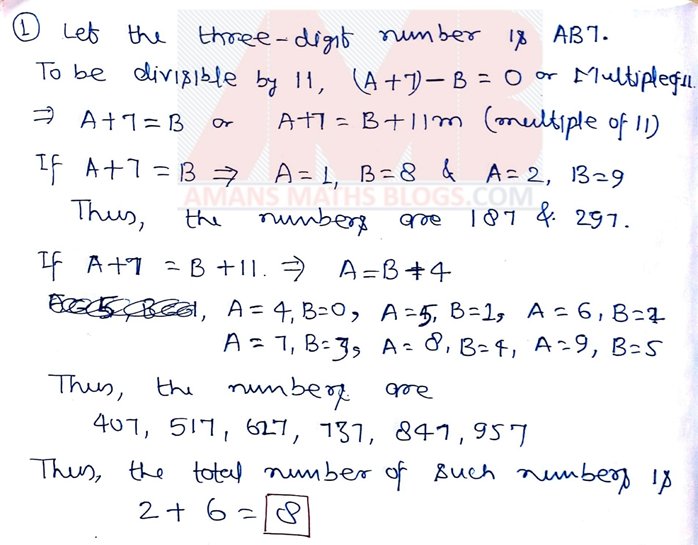Question 2 :

In a six digit number 5 digits are prime numbers. The sum of all the digits is 24. The 2nd, 3rd and 5th digits are identical and the others are distinct digits. The number is divisible by 4. The last digit of the number is

(A) 2 or 4

(B) 4 or 6

(C) 4 or 6 or 8

(D) 2 or 6 or 8

Solution 2 :### NMTC 2007 Stage 1 Primary Level Questions Papers With Solutions

Question 1 :

The natural numbers are written as below following some rule 1, 3, 6, 10, …. The tenth number is

(A) 55

(B) 62

(C) 105

(D) 35

Solution 1 :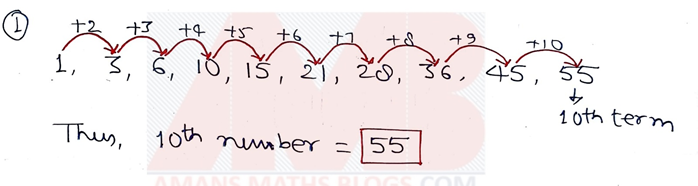Question 2 :

In the adjoining figure, we have 16 dots equally spaced in 4 x 4 grid. From A to B, one has to go. He can go up or down, left or right from one dot to other. In the figure, the length of the path is 7. The maximum length of the path is(A) 12

(B) 13

(C) 15

(D) 16

Solution 2 :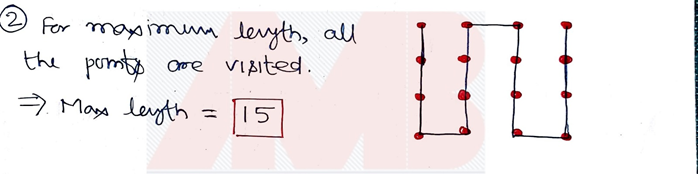### NMTC 2008 Stage 1 Primary Level Questions Papers With Solutions

Question 1 :

In the adjacent figure, ABCD is a square of side 2008 cm. It is divided as shown in the figure into four equal squares. The top right hand side square is again divided into 4 equal squares. Again the right hand square is divided into four equal squares. The side of each smallest square is(A) 1004 cm

(B) 1002 cm

(C) 502 cm

(D) 251 cm

Solution 1 :Question 2 :

Each small square in the figure has a side length 1 cm. An ant travel from P to R. If it moves only along the lines, in how many ways can it reach R, using only the shortest route?(A) 1

(B) 3

(C) 4

(D) 6

Solution 2 :### NMTC 2009 Stage 1 Primary Level Questions Papers With Solutions

Question 1 :

There are 2009 equispaced points on a circle. The number of diameter determined by these points (the extremities of any diameter should be two of these 2009 points) are

(A) 0

(B) 2008

(C) 1004

(D) 2009

Solution 1 :Question 2 :

A 2009 digit number is multiplied by 54. The last two digits of the product are 6 and 8 in this order. If the same number is multiplied by 46, the last two digits are

(A) 0, 9

(B) 8, 6

(C) 3, 2

(D) Cannot be Determined

Solution 2 :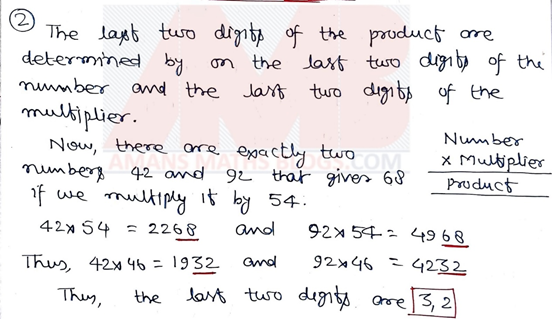### NMTC 2010 Stage 1 Primary Level Questions Papers With Solutions

Question 1 :

n, a are natural numbers each greater than 1. If a + a + a + a + … + a = 2010, and there are n terms on the left hand side, then the number of ordered pairs (a, n) is

(A) 7

(B) 8

(C) 14

(D) 16

Solution 1 :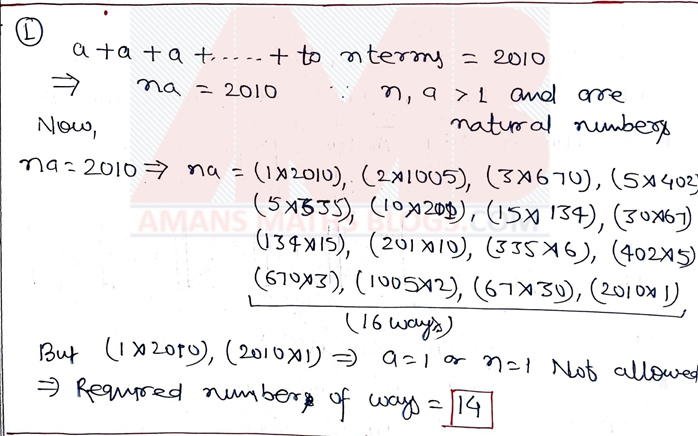Question 2 :

X is a even digit number. Y is an eight digit number 5 more than X. The number of possible values of Y is

(A) 5

(B) 4

(C) 1

(D) 3

Solution 2 :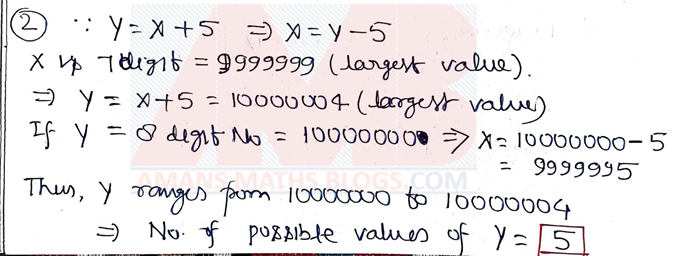### NMTC 2011 Stage 1 Primary Level Questions Papers With Solutions

Question 1 :

When a number n is divided by 10000, the quotient is 1 and the remainder is 2011. The quotient and the remainder when n is divided by 2011 are respectively.

(A) 4, 1936

(B) 5, 1956

(C) 4, 0

(D) 5, 0

Solution 1 :Question 2 :

Baskar is older than Prakash by one year minus one day. Baskar was born on January 1, 2005. The date of birth of Prakash is

(A) January 2, 2006

(B) December 31, 2005

(C) December 31, 2004

(D) January 2, 2004

Solution 2 :### NMTC 2012 Stage 1 Primary Level Questions Papers With Solutions

Question 1 :

a, b where a > b are natural numbers which less than 10 such that (a2 – b2) is a prime number. The number of such pairs (a, b) is

(A) 5

(B) 6

(C) 7

(D) 8

Solution 1 :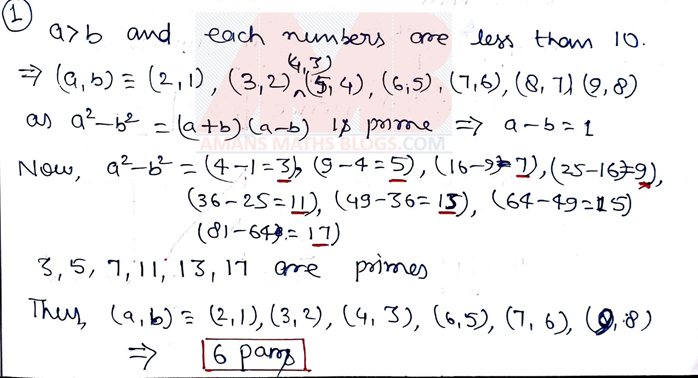Question 2 :

The number of three digit numbers that are divisible by 2 but not divisible by 4 is

(A) 200

(B) 225

(C) 250

(D) 450

Solution 2 :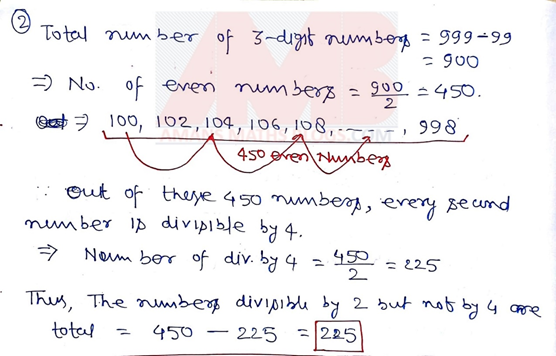### NMTC 2013 Stage 1 Primary Level Questions Papers With Solutions

Question Paper of NMTC 2013 Stage 1 and 2 for Primary Level (Class 5 and 6) is NOT available, so its solutions in NOT available in NMTC 2004 to 2019 Primary Level Questions Papers with Solutions: Click Here

### NMTC 2014 Stage 1 Primary Level Questions Papers With Solutions

Question 1 :

When 26 is divided by a positive integer n, the remainder is 2. The sum of all the possible values of n is

(A) 57

(B) 60

(C) 45

(D) 74

Solution 1 :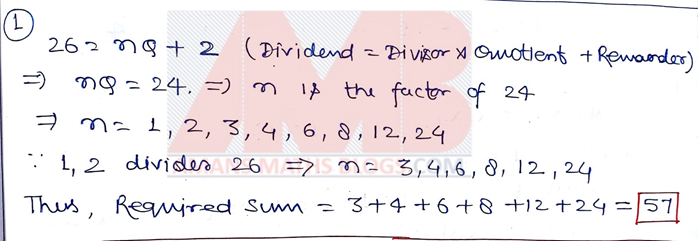Question 2 :

Mahadevan used his calculator (which he rarely uses) to multiply a number by 2. But by mistake he multiplied by 20. To obtain the correct result he must

(A) Divided by 20

(B) Divided by 40

(C) Multiplied by 10

(D) Multiplied by 0.1

Solution 2 :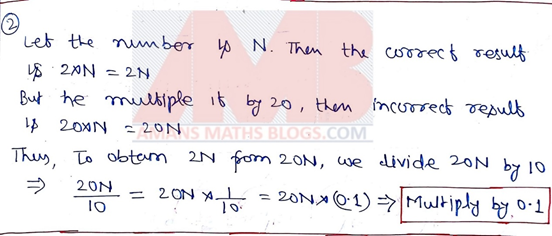### NMTC 2015 Stage 1 Primary Level Questions Papers With Solutions

Question 1 :

A 3-digit number is divisible by 35. The greatest such number has in its tens place the digit

(A) 4

(B) 7

(C) 9

(D) 8

Solution 1 :Question 2 :

When 22015 is completely calculated the units place of the number obtained is

(A) 2

(B) 4

(C) 8

(D) 6

Solution 2 :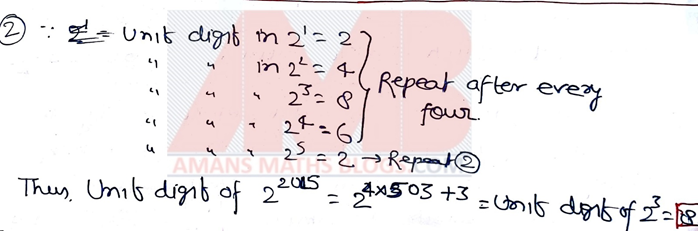### NMTC 2016 Stage 1 Primary Level Questions Papers With Solutions

Question 1 :

The price of an item is decreased by 25%. The percentage increase to be done in the new price to get the original price is

(A) 25%

(B) 30%

(C) 43%

(D) 33 1/3%

Solution 1 :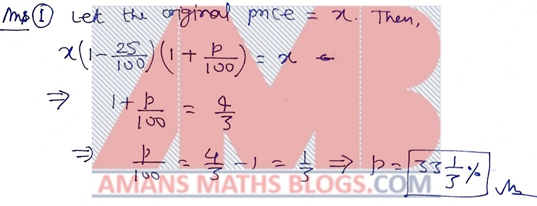Question 2 :

How many pairs of positive integers are there such that their sum is 528 and their HCF is 33?

(A) 4

(B) 6

(C) 8

(D) 12

Solution 2 :### NMTC 2017 Stage 1 Primary Level Questions Papers With Solutions

Question 1 :

Which one of the following numbers in NOT the sum of two prime numbers?

(A) 24

(B) 30

(C) 67

(D) 21

Solution 1 :Question 2 :

ABCD is square and PB = 2AP. The perimeter of the rectangle APQD is 80 cm. The perimeter of ABCD in cm is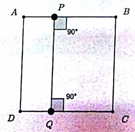(A) 100

(B) 120

(C) 140

(D) 160

Solution 2 :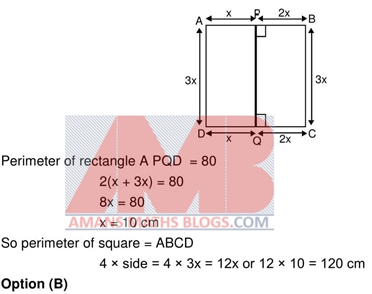### NMTC 2018 Stage 1 Primary Level Questions Papers With Solutions

Question 1 :

Observe the following sequence. What is the 100th term? 7, 8, 1, 0, 0, 1, 0, 1, 1, 0, 2, 1, 0, 3, …

(A) 1

(B) 0

(C) 2

(D) 3

Solution 1 :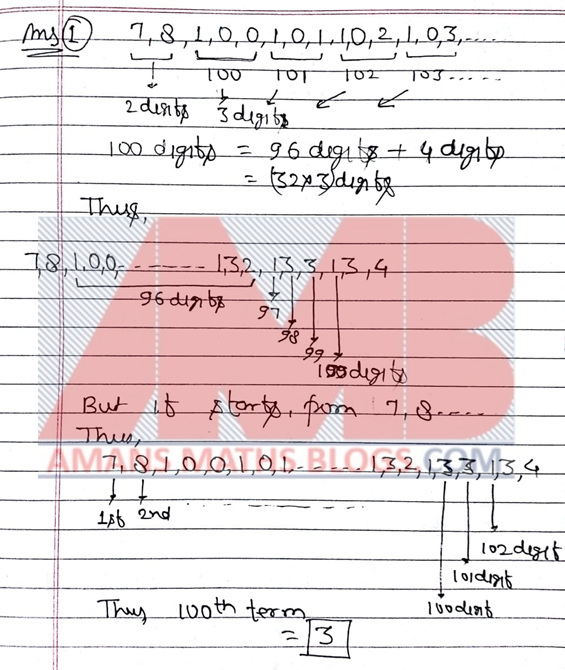Question 2 :

A number is multiplied by 3 then by 1/3, then by 4, then by 1/5 then by 6 and finally by 1/7. The answer is 16. Then the number is

(A) Odd

(B) Even

(C) A Square

(D) A Cube

Solution 2 :### NMTC 2019 Stage 1 Primary Level Questions Papers With Solutions

Question 1 :

How many glasses of 120 mililitres can you fill from a 3 litre can of juice?

(A) 20

(B) 24

(C) 25

(D) 60 ml is left in the can after filing as many glasses as possible.

Solution 1 :Question 2 :

The sum of 2211 + 2213 + 2215 + 2217 + 2219 + 2221 + 2225 + 2227 + 2229 is

(A) 22200

(B) 22225

(C) 22250

(D) 22275

Solution 2 :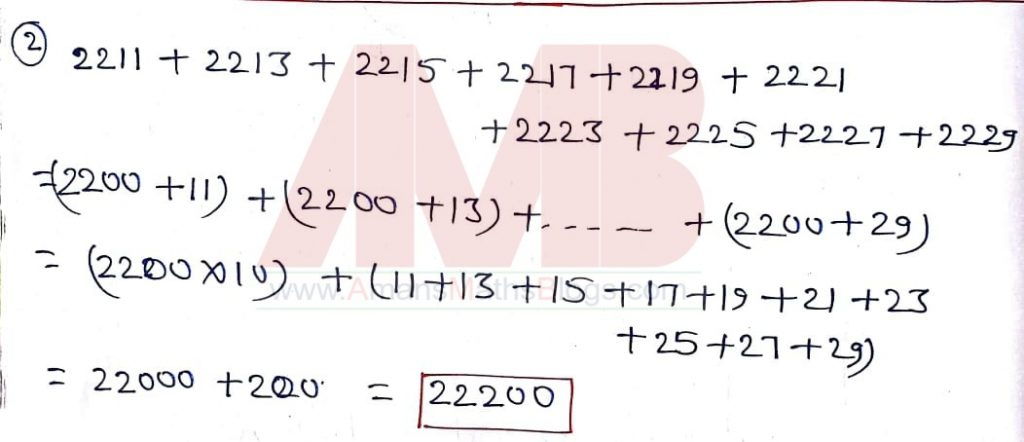## NMTC Stage 2 Primary Level Previous Years Questions Papers with Solutions

After clearing the NMTC stage 1 exam You need to start the preparation for NMTC Stage 2 Primary level exam. For this, you need NMTC Stage 2 Primary Level Previous Years Questions Papers with Solutions. So these papers are given below.

### NMTC 2004 Stage 2 Primary Level Questions Papers With Solutions

Question 1 :

Find all the three digit numbers formed by 3, 5 and 7 in which no digit is repeated. For example, if you do the same for 1, 2, 3 we have 123, 231, 213 as some of the numbers that you can get. Add all of them and divide the sum by 3 + 5 + 7. Call the number that you get as a. Now find all the three digit numbers that are formed by 2, 6 and 8 again without repetitions. Add all of them and divide the sum by 2 + 6 + 8. Call the number as you get as b. Compare a and b to find which is bigger.

Solution 1 :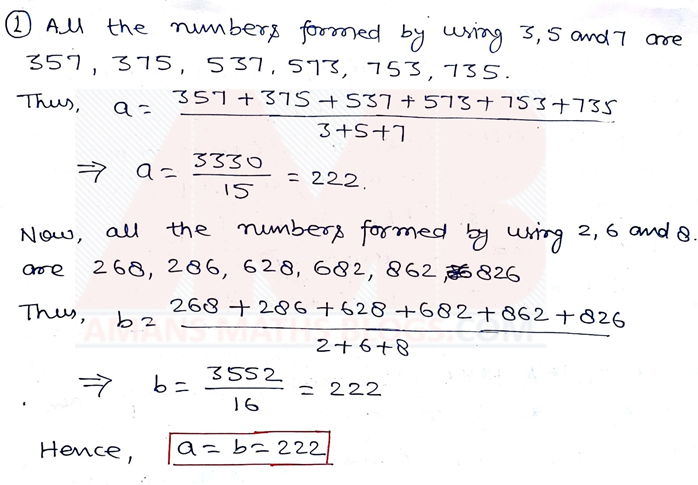### NMTC 2005 Stage 2 Primary Level Questions Papers With Solutions

Question 1 :

Observe the sequence 9, 91, 19, 911, 191, 119, 9111, 1911, 1191, 1119,…What is the 45th term of the sequence?

Solution 1 :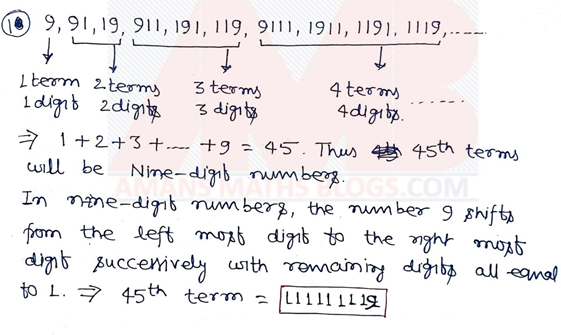### NMTC 2006 Stage 2 Primary Level Questions Papers With Solutions

Question 1 :

In a pair of dice one die has 3 one and 3 fours on its faces. What should be the numbers on the faces of other die so that the possible sums of the numbers shown on the dice are from 1 to 12 when both are rolled? (For example: if one die has 0, 0, 0, 1, 1, 1) on its faces and the other die has 1, 3, 5, 7, 9, 11 then some of the possible sums are 0 + 1 = 1, 1 + 7 = 8, 1 + 11 = 12 etc.)

Solution 1 :### NMTC 2007 Stage 2 Primary Level Questions Papers With Solutions

Question 1 :

Find all natural numbers which divide 2007 and leave a remainder of 207.

Solution 1 :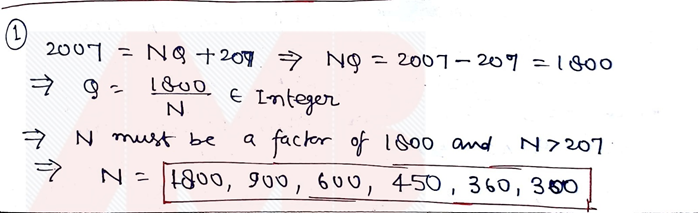### NMTC 2008 Stage 2 Primary Level Questions Papers With Solutions

Question 1 :

A three digit number has the following properties.

• It is divisible by 5.
• None of the digits is zero.
• When the number is written in the reverse order it is divisible by 6.

Find all such three digit numbers. Find their sum and all the prime divisors of the sum.

Solution 1 :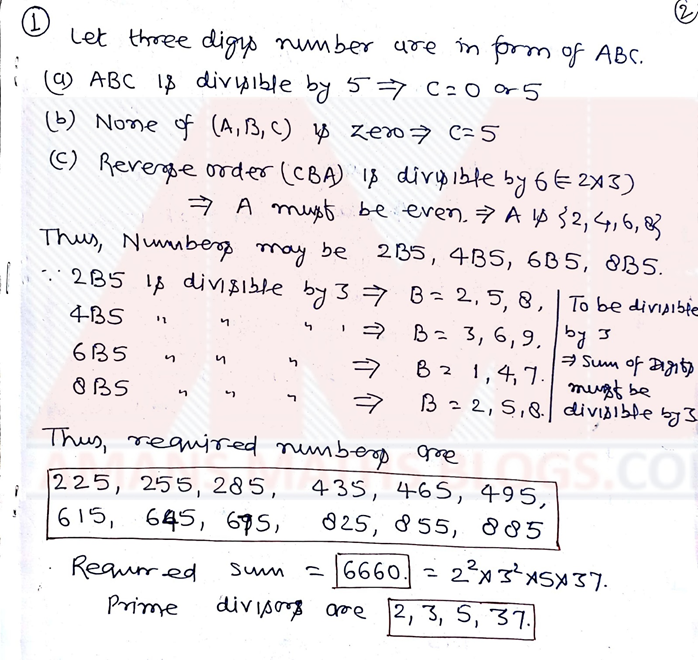### NMTC 2009 Stage 2 Primary Level Questions Papers With Solutions

Question 1 :

Find the total number of digits in the number 1234…200720082009.

Solution 1 :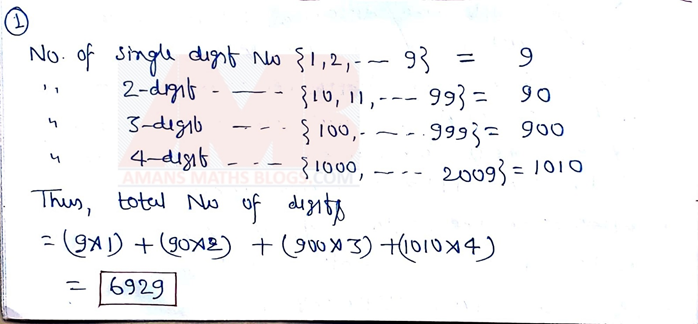### NMTC 2010 Stage 2 Primary Level Questions Papers With Solutions

Question 1 :

Given two numbers say 1 and 2, the addition problem is 1 + 2 (or 2 + 1). Thus, there is just one addition problem. (Considering 1 + 2 and 2 + 1 are same). Given three numbers 1, 2 and 3. The addition of the problems are 1 + 2 + 3 = 6, 12 + 3, 21 + 3, 31 + 2, 13 + 2, 32 + 1, 23 + 1. Thus, there are seven addition problems. Using all the four numbers 1,2,3,4 list all the two-digit addend addition problems. Find the sum in each case. What is the maximum sum and minimum sum in each case.

Solution 1 :### NMTC 2011 Stage 2 Primary Level Questions Papers With Solutions

Question 1 :

Pustak Keeda of standard six bought a book. On the first day he reads one fifth of the number of pages of the book plus 12 pages. On the second day, he reads one-fourth of the remaining pages plus 15 pages and on the third day he read one-third of the remaining pages plus 20 pages.  The fourth day which is the final day he read the remaining 60 pages of the book and complete the reading. Find the total number of pages in the book and the number of pages read on each day.

Solution 1 :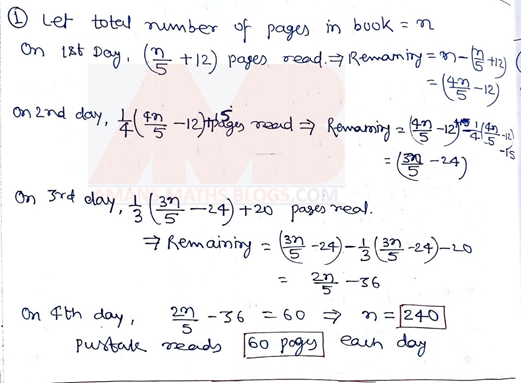### NMTC 2012 Stage 2 Primary Level Questions Papers With Solutions

Question 1 :

Find the sum (S) of all the numbers with 2012 digits and digital sum 2. Also, find the digital sum of S.

Solution 1 :### NMTC 2013 Stage 2 Primary Level Questions Papers With Solutions

Question Paper of NMTC 2013 Stage 1 and 2 for Primary Level (Class 5 and 6) is NOT available, so its solutions in NOT available in NMTC 2004 to 2019 Primary Level Questions Papers with Solutions: Click Here

### NMTC 2014 Stage 2 Primary Level Questions Papers With Solutions

Question 1 :

A three-digit number is divisible by 7 and 8.

• How many such number are there?
• List out all the numbers.
• Spot out the two numbers whose digits um is maximum and minimum.

For how many numbers the digit sum is odd?

Solution 1 :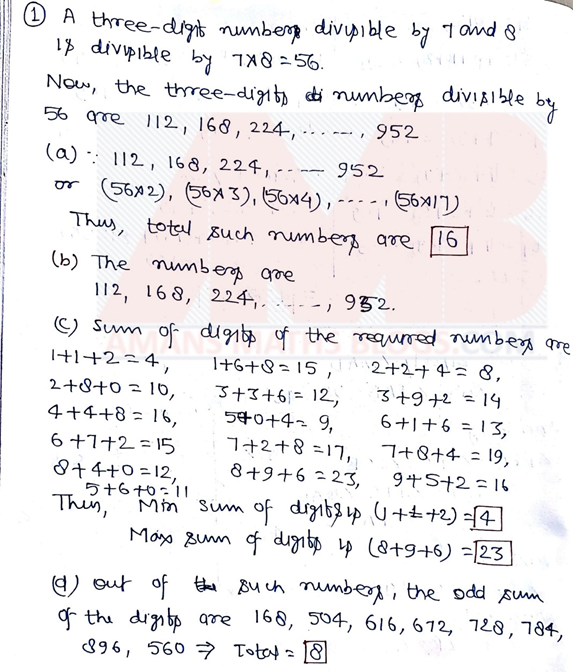### NMTC 2015 Stage 2 Primary Level Questions Papers With Solutions

Question 1 :

A unit fraction is one of the form 1/a, where a is a natural number and not equal to 1. Any proper fraction can be written as the sum of two or more unit fractions. {Example: 1/2 = 1/3 + 1/6, 5/6 = 1/2 + 1/3, 1/24 = 1/54 + 1/72 + 1/108}. In the case of 1/2, the factors of 2 are 1 and 2 only. 1/2 = (1 + 2)/(2(1 + 2)) = 1/2(1 + 2) + 2/2(1 + 2) = 1/6 + 1/3. Express 1/15 as the sum of two different unit fraction in 2 different ways.

Solution 1 :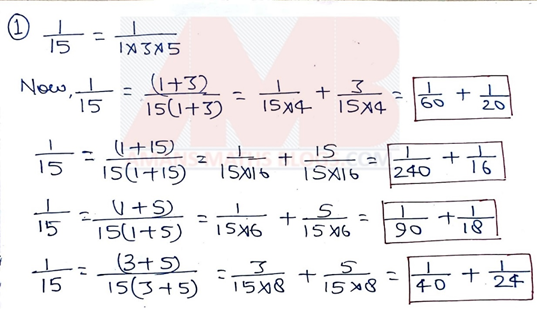### NMTC 2016 Stage 2 Primary Level Questions Papers With Solutions

Question 1 :

A positive integer n has five digits. N is the six-digit number obtained by adjoining 2 as the leftmost digit of n. M is the six-digit number by adjoining 2 at right most digit of n. If M = 3N, find all the values of n

Solution 1 :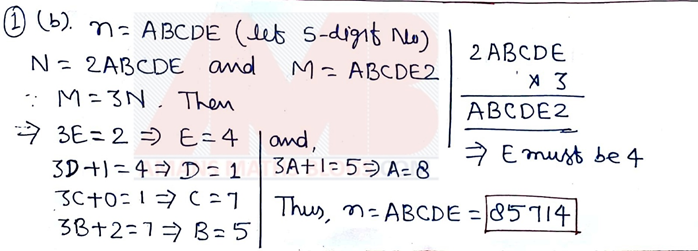### NMTC 2017 Stage 2 Primary Level Questions Papers With Solutions

Question 1 :

If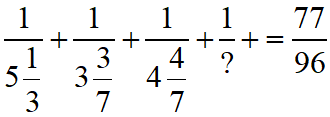, find what should be filled in the place marked?

Solution 1 :### NMTC 2018 Stage 2 Primary Level Questions Papers With Solutions

Question 1 :

If Write down all the ten digit numbers whose digital sum is 2. (The digital sum of a number is the sum of the digits of the number. The digital sum of 4022 is 4 + 0 + 2 + 2 is 8). Find the sum of all the 10 digits numbers with digital sum 2.

Solution 1 :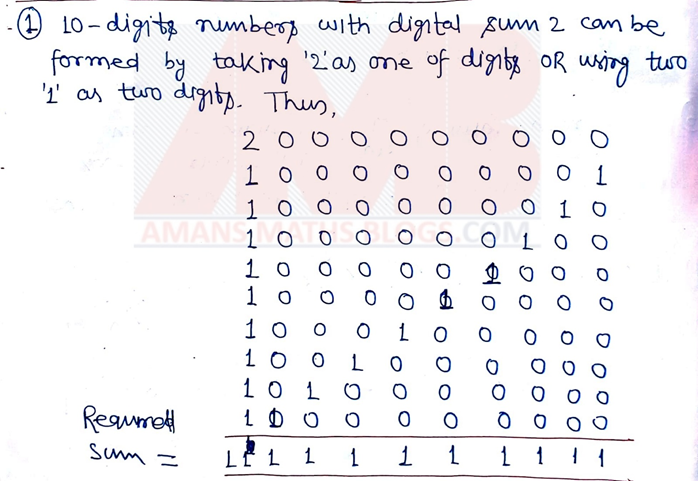### NMTC 2019 Stage 2 Primary Level Questions Papers With Solutions

Question 1 :

(a) Find the positive integers m, n such that 1/m + 1/n = 3/17

(b) Find the positive integers m, n and p such that 1/m + 1/n + 1/p = 3/17

Solution 1 :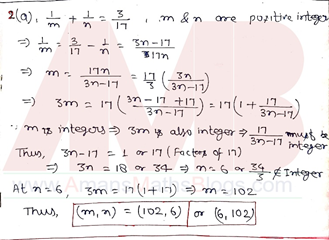## Get NMTC Primary Level Previous Years Papers with Solutions EBook

To get the EBook PDF of NMTC 2004 to 2019 Stage 1 and 2 for Primary Level Questions Papers With Answer Keys & Solutions, Click on Image Below.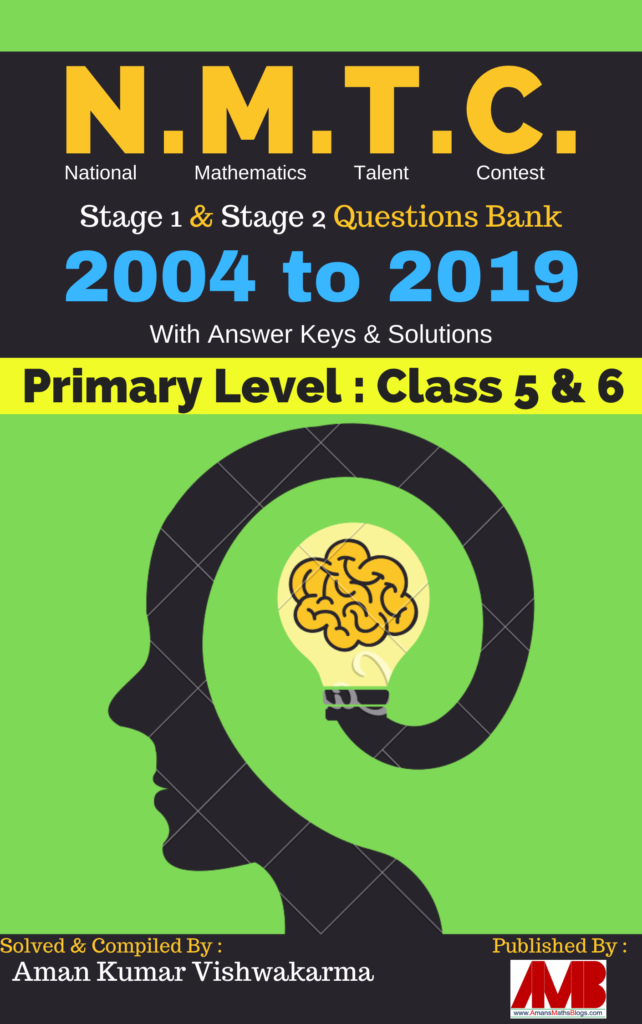## NMTC Stage 1 & 2 Sub Junior Level Previous Years Questions Papers with Solutions

Since NMTC exams is categorized into five levels according to class/grade studying the students, NMTC Sub-Junior Level exam is known as Kaprekar Contest, its eligibility is for Class 7 and 8. Thus, to prepare for NMTC Sub Junior level exam, you need NMTC Stage 1 & 2 Sub Junior Level Previous Years Questions Papers with SolutionsClick Here : NMTC 2004 to 2019 Sub-Junior Level Stage 1 & 2 Previous Years Papers with Solutions.

## NMTC Stage 1 & 2 Junior Level Previous Years Questions Papers with Solutions

NMTC Junior Level exam is known as Bhaskar Contest, its eligibility is for Class 7 and 8. Thus, to prepare for NMTC Junior level exam, you need NMTC Stage 1 & 2 Junior Level Previous Years Questions Papers with Solutions. The EBook PDF of NMTC Stage 1 & 2 Junior Level Previous Years Questions Papers with Solutions will be released in next session 2021-22.

error: Content is protected !!